# Poisson stability

(diff) ← Older revision | Latest revision (diff) | Newer revision → (diff)

The property of a point(a trajectory) of a dynamical system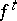(or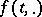, cf. ), given in a topological space, consisting in the following: There are sequences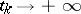,such that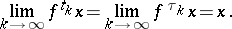In other words,is an- and-limit point (cf. Limit point of a trajectory) of the trajectory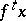. The concept of Poisson stability was introduced by H. Poincaré  on the basis of an analysis of results of Poisson on the stability of planetary orbits.

Every Poisson-stable point is non-wandering; the converse is not true (cf. Wandering point). Every fixed and every periodic point, more generally, every recurrent point, is Poisson stable. Ifand the dynamical system is smooth (i.e. given by a vector field of class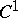), then every Poisson-stable point is either fixed or periodic (cf. Poincaré–Bendixson theory).

Poincaré's recurrence theorem (cf. Poincaré return theorem): If a dynamical system is given in a bounded domain ofand if Lebesgue measure is an invariant measure of the system, then all points are Poisson stable, with the exception of a certain set of the first category of measure zero (cf. , ). A generalization of this theorem to dynamical systems given on a space of infinite measure is the Hopf recurrence theorem (cf. ): If a dynamical system is given on an arbitrary domain in(e.g. onitself) and if Lebesgue measure is an invariant measure of the system, then every point, with the exception of the points of a certain set of measure zero, is either Poisson stable or divergent, i.e.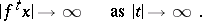There are still more general formulations of the theorems of Poincaré and E. Hopf (cf. ).

How to Cite This Entry:
Poisson stability. Encyclopedia of Mathematics. URL: http://encyclopediaofmath.org/index.php?title=Poisson_stability&oldid=12373
This article was adapted from an original article by V.M. Millionshchikov (originator), which appeared in Encyclopedia of Mathematics - ISBN 1402006098. See original article